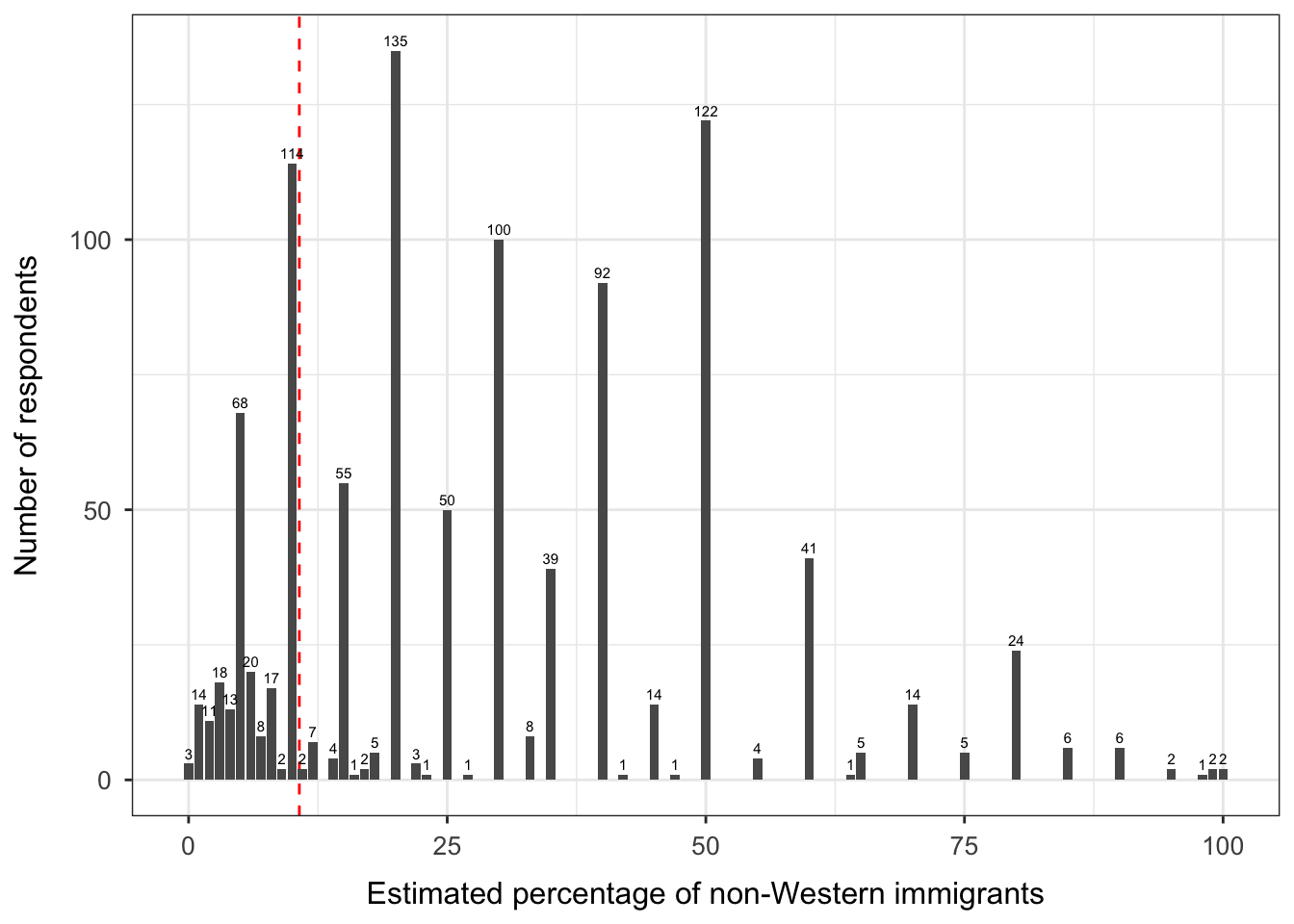## Data

We will use data from the 2009 British Social Attitudes Survey. You can download the data here. The codebook is available here.

Our goal is to predict the following output variable (the name in brackets corresponds to the variable name in the codebook):

• imm_brit [IMMBRIT]: Out of every $$100$$ people in Britain, how many do you think are immigrants from non-western countries?

We will use the following input variables:

• resp_female [RSex]: Is the respondent female?
• resp_age [RAge]: Age of the respondent
• resp_household_size [Househld]: How many people live in respondent’s household?
• resp_party_cons [PartyIDN]: Respondent is most likely to support the Conservative Party
• resp_party_lab [PartyIDN]: Respondent is most likely to support the Labour Party
• resp_party_libdem [PartyIDN]: Respondent is most likely to support the Liberal Democratic Party
• resp_party_snp [PartyIDN]: Respondent is most likely to support the Scottish National Party
• resp_party_green [PartyIDN]: Respondent is most likely to support the Green Party
• resp_party_ukip [PartyIDN]: Respondent is most likely to support the UK Independence Party
• resp_party_bnp [PartyIDN]: Respondent is most likely to support the British National Party
• resp_party_other [PartyIDN]: Respondent is most likely to support another party, no party, refused to say, or did not know
• resp_newspaper [Readpap]: Respondent normally reads a daily newspaper
• resp_internet_hrs [WWWHrsWk]: Hours the respondent spends using the internet (per week)
• resp_religious [Religion]: Respondent regards himself/herself belonging to a particular religion
• resp_time_current_employment [EmploydT]: Months respondent has spent with current employer
• resp_urban_area [PopBand]: Population density
• resp_health [SRHealth]: Respondent’s health
• resp_household_income [HHincome]: Total income of respondent’s household

Let’s have a look at the distribution of the output variable. The red dashed line shows the true percentage of non-Western immigrants (see www.migrationwatchuk.org).The plot shows that 756 of 1,044 respondents (or 72.4%) overestimate the percentage of non-Western immigrants in the UK.

## Data Preparation

The original data set contains 1,044 respondents.

library(foreign)
library(dplyr)

# Set working directory
# setwd(...)

# Load data set
load("bsas_short.RData")

# Declare factor variables
bsas_data <- bsas_data %>%
dplyr::mutate(resp_urban_area = factor(resp_urban_area,
levels = 1:4,
labels = c("rural", "rather rural",
"rather urban", "urban")),
resp_health = factor(resp_health,
levels = 0:3,
labels = c("bad", "fair", "fairly good", "good")))

We will use the glmnet() function in the glmnet package to perform ridge regression and the lasso. Before doing so, we use the model.matrix() function to create a matrix of input variables. This function automatically transforms any qualitative variables into dummy variables. This is important because glmnet() can only take quantitative inputs.

We remove the intercept from the matrix produced by model.matrix() because glmnet() will automatically include an intercept. We also exclude the input resp_party_cons, which will serve as the baseline in our model.

# Matrix of input variables (remove the intercept and resp_party_cons)
x <- model.matrix(imm_brit ~ . -1 -resp_party_cons, bsas_data)

# Output variable
y <- bsas_data\$imm_brit

## Exercise 1: Ridge Regression

The goal of the first exercise is to fit a ridge regression to our data.

### Problem 1.1

1. Split the data into a training set and a test set.
2. Define a vector of values for the tuning parameter $$\lambda$$. Use the training set to fit a ridge regression for each $$\lambda$$ value. You can do this by using the glmnet() function in the glmnet package. The glmnet() function has an alpha argument that determines what type of model is fit. If alpha = 0, then a ridge regression model is fit, and if alpha = 1, then a lasso model is fit.
3. Use the plot() function to create a graph that shows the shrinkage of the coefficient estimates as a function of $$\lambda$$.

### Problem 1.2

1. Perform cross-validation on the trainig set to choose the optimal tuning parameter $$\lambda$$. You can do this by using the cross-validation function cv.glmnet() in the glmnet package. Use the plot() function to create a graph that shows the CV estimate of the expected test MSE associated with each value of $$\lambda$$.
2. Find the optimal value of $$\lambda$$. Use the test set to estimate the test error associated with the optimal model.
3. Re-fit the ridge regression model on the full data set, using the optimal value of $$\lambda$$.

## Exercise 2: The Lasso

The goal of the second exercise is to fit a lasso model to our data.

### Problem 2.1

1. Use the training set to fit a lasso model for each $$\lambda$$ value. You can again use the glmnet() function, setting the argument alpha = 1. Use the plot() function to create a graph that shows the shrinkage of the estimated coefficients as a function of $$\lambda$$.
2. Perform cross-validation on the training set to find the optimal value of $$\lambda$$. Plot the CV error associated with each value of $$\lambda$$ and use the test set to estimate the test error for the optimal model.
3. Re-fit the lasso model on the full data set, using the optimal value of $$\lambda$$.